# 【数据结构与算法】-（1）基础篇

## 一、数据结构

### 2 术语概念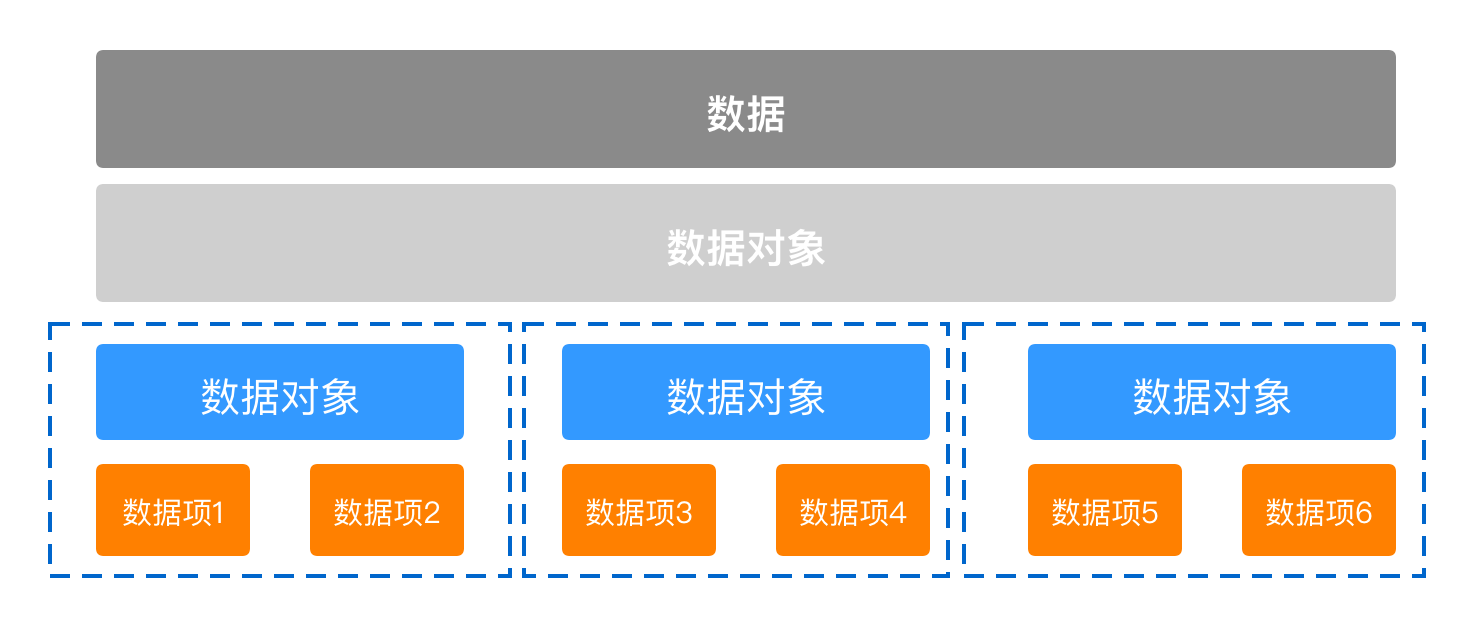``````//声明一个结构体类型
struct Teacher{     //一种数据结构
char *name;     //数据项--名字
char *title;    //数据项--职称
int  age;       //数据项--年龄
};

int main(int argc, const char * argv[]) {

struct Teacher t1;     //数据元素;
struct Teacher tArray; //数据对象;

return 0;
}``````

——《大话数据结构》

• 可以输入到计算机
• 可以被计算机程序处理

### 3 逻辑结构与物理结构

#### 3.1 逻辑结构

##### 1.3.1 线性结构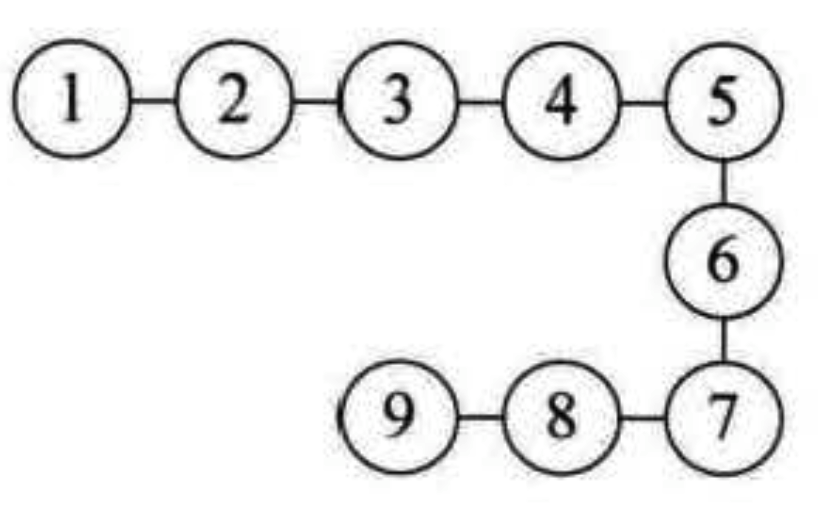1. 线性表
2. 栈和队列
3. 字符串
##### 1.3.2 非线性结构

1. 集合结构
集合结构中的数据元素除了同属于一个集合外，他们之间没有其他关系。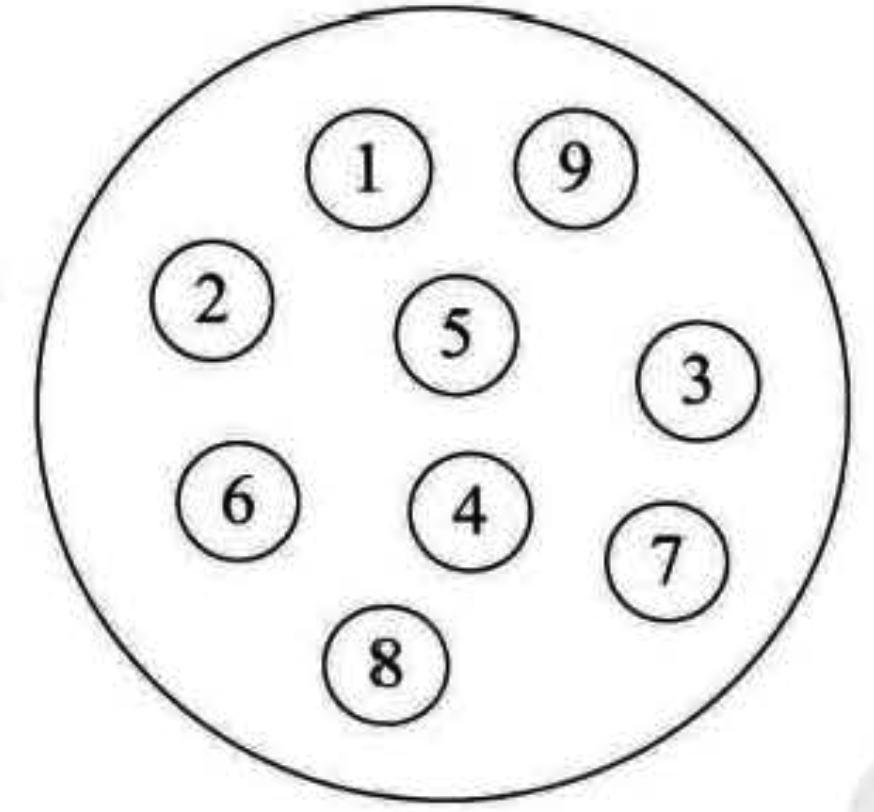2. 树形结构
树形结构中的数据元素之间存在一种一对多的层次关系。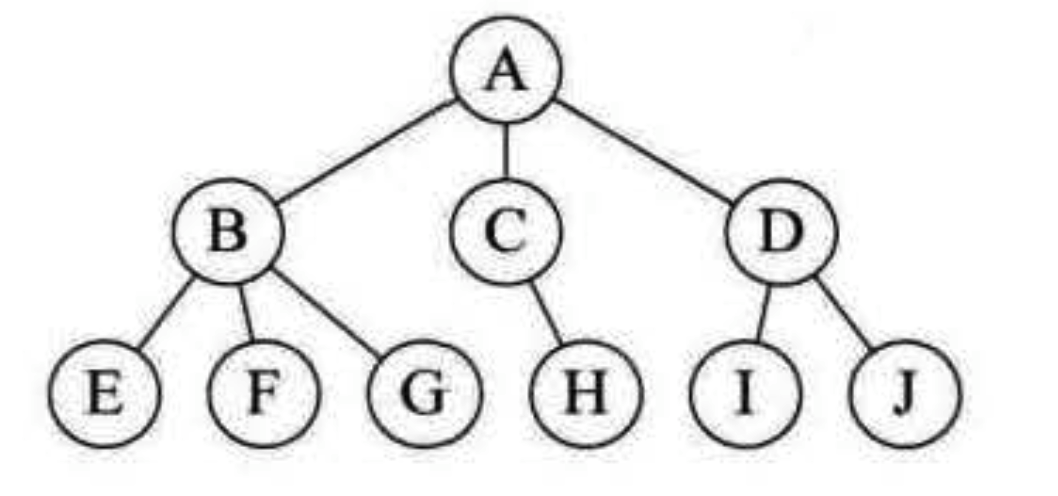3. 图形结构
图形结构中的数据元素是多对多的关系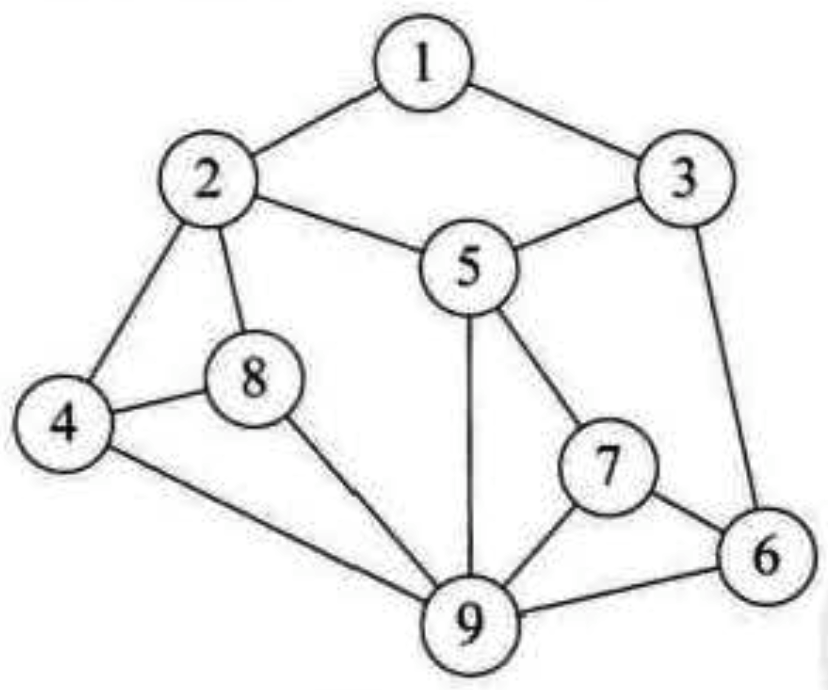#### 3.2 物理结构

##### 3.2.1 顺序存储结构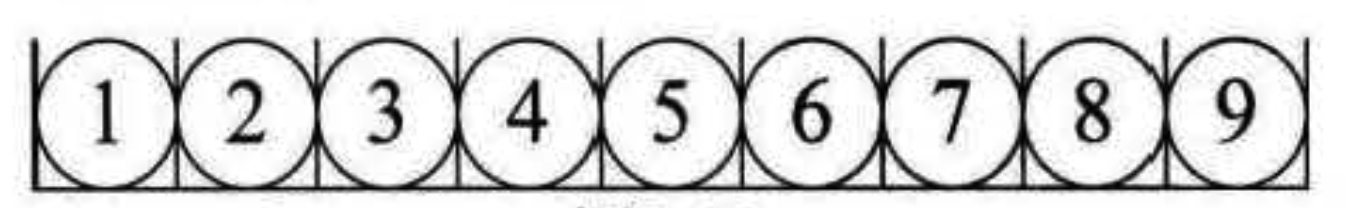##### 3.2.2 链式存储结构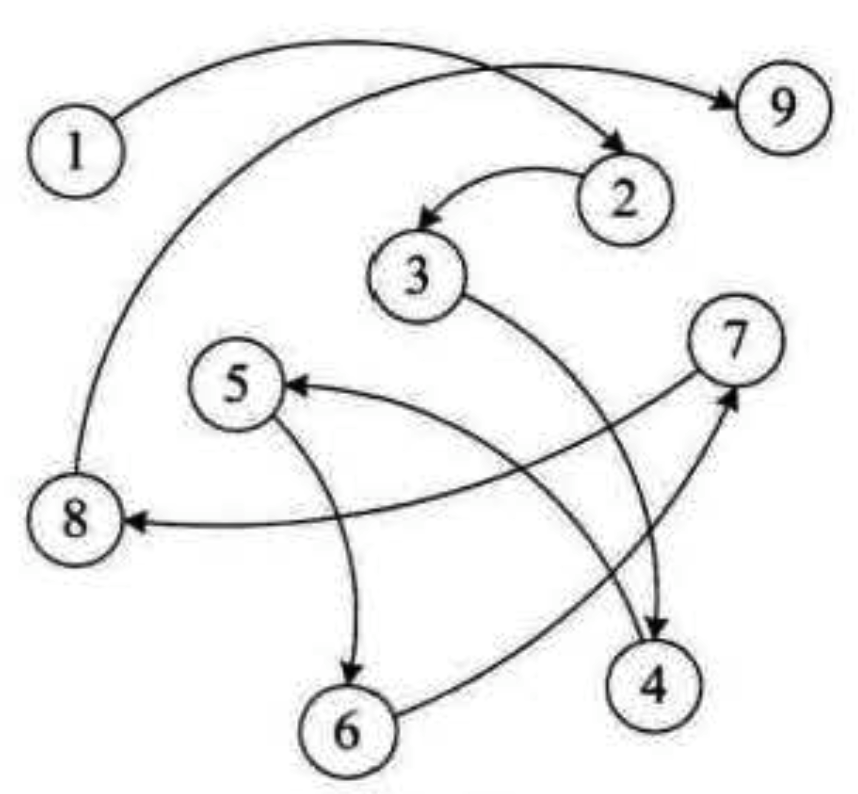### 4 抽象数据类型

#### 4.1 数据类型

• 原子类型：不可以再分解的基本类型。包括整型、浮点型、字符类型等
• 结构类型：由若干个类型组合而成，是可以再分解的。例如，整型数组是由若干整型数据组成的。

## 二、算法

### 2.2 特性

1. 有穷性
指算法在执行有限的步骤之后，自动结束而不会出现无限循环，而且每一个步骤在可接受的时间内完成。
2. 确定性
算法的每一步骤都具有确定的含义，不会出现二义性。
3. 可行性
算法的每一步都必须是可行的，也就是说，每一步都能通过执行有限次数完成
4. 输入输出
算法具有零个或多个输入

### 2.3 算法设计的要求

#### 2.3.1 正确性

1. 算法程序没有语法错误。
2. 算法程序对于合法的输入数据能够产生满足要求的输出结果。
3. 算法程序对于非法的输入数据能够得出满足规格说明的结果。
4. 算法程序对于精心选择的，甚至刁难的测试数据都有满足要求的输出结果。

### 2.4 效率的度量方法

#### 2.4.2 事前统计方法

1. 算法采用的策略、方法
2. 编译产生的代码质量
3. 问题的输入规模
4. 机器执行指令的速度

• 第一种算法

``````int i, sum = 0 n = 100;                        /* 执行 1次*/
for(i = 1; i <= n; i++)                        /* 执行 n + 1 次*/
{
sum += i;                                            /* 执行 n 次*/
}
print("%d", sum);                                    /* 执行 1 次*/``````
• 第二种算法

``````int sum = 0, n = 100;                            /* 执行 1次*/
sum = (1 + n) * n/2;                            /* 执行 1次*/
printf("%d", sum);                                /* 执行 1次*/``````

### 2.5 算法时间复杂度

#### 2.5.2 推导大O 阶方法

1. 用常数1 取代运行时间中的所有加法常数
2. 在修改后的运行次数函数中，只保留最高阶项。
3. 如果最高阶项存在且不是1，则去除与这个项相乘的常数

#### 2.5.3 常数阶

``````int sum = 0, n = 100;                            /* 执行 1次*/
sum = (1 + n) * n/2;                            /* 执行 1次*/
printf("%d", sum);                                /* 执行 1次*/``````

``````int sum = 0, n = 100;                            /* 执行 1次*/
sum = (1 + n) * n/2;                            /* 执行 1次*/
sum = (1 + n) * n/2;                            /* 执行 1次*/
sum = (1 + n) * n/2;                            /* 执行 1次*/
sum = (1 + n) * n/2;                            /* 执行 1次*/
sum = (1 + n) * n/2;                            /* 执行 1次*/
sum = (1 + n) * n/2;                            /* 执行 1次*/
sum = (1 + n) * n/2;                            /* 执行 1次*/
sum = (1 + n) * n/2;                            /* 执行 1次*/
sum = (1 + n) * n/2;                            /* 执行 1次*/
sum = (1 + n) * n/2;                            /* 执行 1次*/
printf("%d", sum);                                /* 执行 1次*/``````

#### 2.5.4 线性阶

``````int i;
for(i = 0; i < n; i++)
{
/ *
时间复杂度为O（1）的程序步骤序列
*/
}``````

#### 2.5.5 对数阶

``````int count = 1;
while (count < n)
{
count = count * 2;
/* 时间复杂度为 O(1) 的程序步骤序列*/
}``````

#### 2.5.6 平方阶

``````in i,j;
for(i = 0; i < n; i++)
{
for (j = 0; j < n; j++)
{
/ * 时间复杂度为 O(1) 的程序步骤序列*/
}
}``````

### 2.6 常见的时间复杂度

12 O(1) 常数阶
2n + 3 O(n) 线性阶
3n2 + 2n + 1 O(n2) 平方阶
5 log2n + 20 O(logn) 对数阶
2n + 3n log2n + 19 O(nlogn) nlogn 阶
6n3 + 2 n2 + 3n + 4 O(n3) 立方阶
2 n O(2n) 指数阶

O(1) < O(logn) < O(n) < O(nlogn) < O(n2) < O(n3) < O(2n) < O(n!) < O(nn)

### 2.8 算法空间复杂度

上一篇【类的加载】-（3）loading_images

2020-04-01 李佳【类的加载】-（2）懒加载类与分类

2020-03-31 李佳
目录